# Variable selection for high-dimensional data

### Chapter 5.3: Stochastic search variable selection

These data come from

Lan H, Chen M, Flowers JB, et al (2006). Combined expression trait correlations and expression quantitative trait locus mapping. PLoS Genetics.

which can be downloaded from http://www.ncbi.nlm.nih.gov/geo (accession number GSE3330). In the study, $$n=60$$ mice (31 female) were sampled and the physiological phenotypes stearoyl-CoA desaturase 1 (SCD1) is taken as the response to be regressed on the expression levels of 22,575 genes. Following Bondell and Reich (JASA, 2012), we use only the $$p=1000$$ genes with highest correlation with the response as predictors in the model.

We use the linear regression model $Y_i \sim \mbox{Normal}(\alpha + \sum_{j=1}^pX_{ij}\beta_j,\sigma^2_e)$ with stochastic search variable selection prior $\beta_j = \gamma_j\delta_j \mbox{ with }\gamma_j \sim \mbox{Bernoulli}(q) \mbox{ and }\delta_j \sim \mbox{Normal}(0,\sigma_e^2\sigma_b^2).$

The code below fits the model with priors $$q\sim\mbox{Beta}(1,1)$$ and $$\sigma^2_e,\sigma_b^2\sim\mbox{InvGamma}(0.1,0.1)$$ and presents the marginal inclusion probabilities $$\mbox{Prob}(\beta_j\ne 0|Y)$$. We also test for sensitivity to the prior by refitting with priors $$q\sim\mbox{Beta}(1,2)$$ and $$\sigma_b^2\sim\mbox{InvGamma}(0.5,0.5)$$.

## Load the data

dat <- read.table("lan_data.txt",header=T)
dat <- t(as.matrix(dat[,-1]))
Y   <- dat[,c(22577,22578, 22579)]
Y   <- Y[,1] # pick the first of the three responses
X   <- scale(dat[,1:22575])
sex <- dat[,22576]
n   <- length(Y)
p   <- ncol(X)

n

##  60

p

##  22575

cor_xy = rep(0,p)
for(i in 1:p){
cor_xy[i] <- abs(cor(X[,i],Y))
}
keep <- rank(-cor_xy)<=1000
boxplot(cor_xy~keep,xlab="Kept in model",ylab="Corr with Y")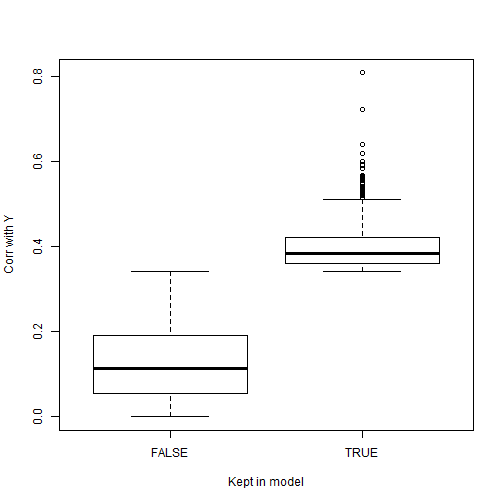X    <- cbind(sex,X[,keep])
p    <- ncol(X)


## Define a Gibbs sampler in R

With $$p=1000$$ covariates JAGS is too slow and so we code our own Gibbs sampler.

SSVS<-function(Y,X,iters=2000,thin=10,
a1=0.1,b1=0.1,a2=0.1,b2=0.1,a3=1,b3=1,eps=0.0001){

n  <- length(Y)
p  <- ncol(X)

#initial values:

alpha <- mean(Y)
taue  <- 1/var(Y)
beta  <- rep(0,p)
gamma <- rep(0,p)
delta <- rep(0,p)
taub  <- 1
q     <- 0.5

#keep track of stuff:
keep.beta  <- matrix(0,iters,p)
R          <- Y-alpha-X%*%beta # Keep track of the residuals

# Start the Gibbs sampler:
for(i in 1:iters){

for(rep in 1:thin){ # Thinning level

# Update the beta's one at a time
for(j in 1:p){
R        <- R + X[,j]*beta[j]
# Continuous part
if(gamma[j]==0){
delta[j] <- rnorm(1,0,1/sqrt(taue*taub))
}
if(gamma[j]==1){
VVV      <- taue*sum(X[,j]^2)+taue*taub
MMM      <- taue*sum(X[,j]*R)
delta[j] <- rnorm(1,MMM/VVV,1/sqrt(VVV))
}

# Indicator part
log.p.in  <- log(q)-0.5*taue*sum((R-X[,j]*delta[j])^2)
log.p.out <- log(1-q)-0.5*taue*sum(R^2)
diff      <- log.p.in-log.p.out
diff      <- ifelse(diff>10,10,diff)
p.in      <- exp(diff)/(1+exp(diff))
gamma[j]  <- rbinom(1,1,p.in)

beta[j]   <- delta[j]*gamma[j]
R         <- R - X[,j]*beta[j]
}

# Update the hyperparameters
taue  <- rgamma(1,(n+p)/2+a1,sum(R^2)/2+taub*sum(delta^2)/2+b1)
taub  <- rgamma(1,p/2+a2,taue*sum(delta^2)/2+b2)
q     <- rbeta(1,sum(gamma)+a3,sum(1-gamma)+b3)
R     <- R+alpha
VVV   <- taue*n+eps
MMM   <- taue*sum(R)
alpha <- rnorm(1,MMM/VVV,1/sqrt(VVV))
R     <- R-alpha
}

#Store the output:
keep.beta[i,] <- beta
}

return(keep.beta)}


## Fit the model and check convergence

   burn  <- 2000
iters <- 10000
thin  <- 20

set.seed(0820)
beta1 <- SSVS(Y,X,a2=0.1,b2=0.1,a3=1,b3=1,iters=iters,thin=thin)

set.seed(0820)
beta2 <- SSVS(Y,X,a2=0.1,b2=0.1,a3=1,b3=2,iters=iters,thin=thin)

set.seed(0820)
beta3 <- SSVS(Y,X,a2=0.5,b2=0.5,a3=1,b3=1,iters=iters,thin=thin)

p1    <- rowSums(beta1!=0)
p2    <- rowSums(beta2!=0)
p3    <- rowSums(beta3!=0)

plot(p1,type="l",col=1,ylim=c(0,p),
xlab="MCMC iteration",ylab="Number of variables in the model")
lines(p2,col=2)
lines(p3,col=3)

legend("topright",paste("Prior",1:3),lty=1,col=1:3,bty="n")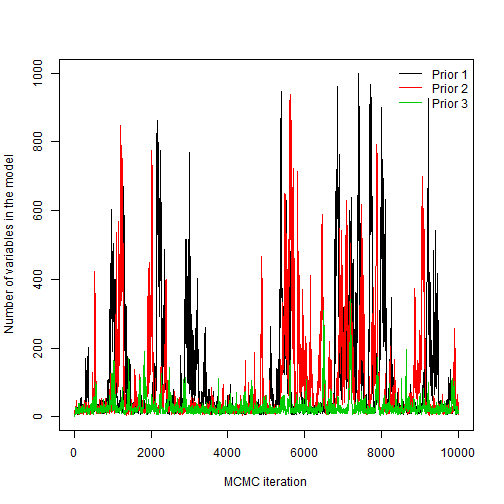## Summarize the marginal inclusion probabilities, $$\mbox{Prob}(\beta_j\ne 0|Y)$$

   Inc_Prob1 <- colMeans(beta1[burn:iters,]!=0)
Inc_Prob2 <- colMeans(beta2[burn:iters,]!=0)
Inc_Prob3 <- colMeans(beta3[burn:iters,]!=0)

plot(Inc_Prob1,Inc_Prob2,xlim=0:1,ylim=0:1,
main="Inclusion probabilities",
xlab="Prior 1", ylab="Prior 2")
abline(0,1)
abline(0.5,0,lty=2)
abline(v=0.5,lty=2)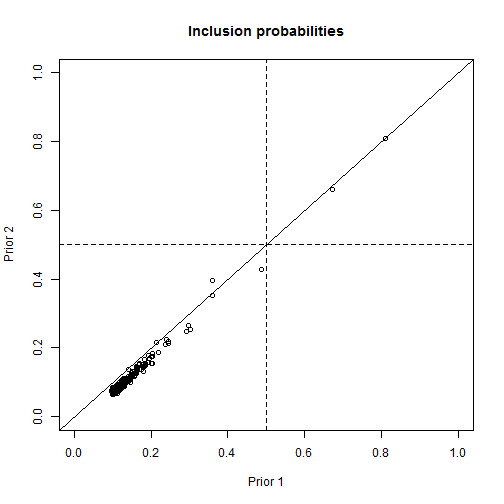plot(Inc_Prob1,Inc_Prob3,xlim=0:1,ylim=0:1,
main="Inclusion probabilities",
xlab="Prior 1", ylab="Prior 3")
abline(0,1)
abline(0.5,0,lty=2)
abline(v=0.5,lty=2)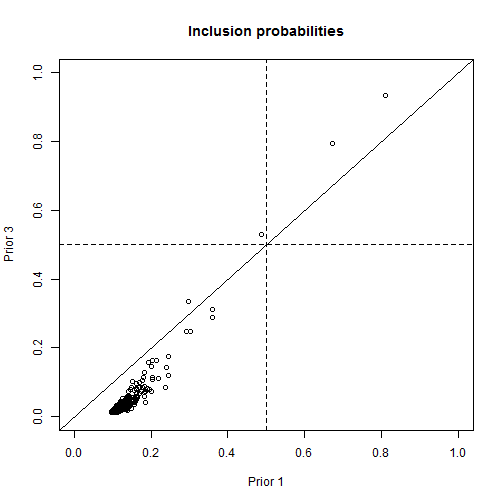plot(Inc_Prob2,Inc_Prob3,xlim=0:1,ylim=0:1,
main="Inclusion probabilities",
xlab="Prior 2", ylab="Prior 3")
abline(0,1)
abline(0.5,0,lty=2)
abline(v=0.5,lty=2)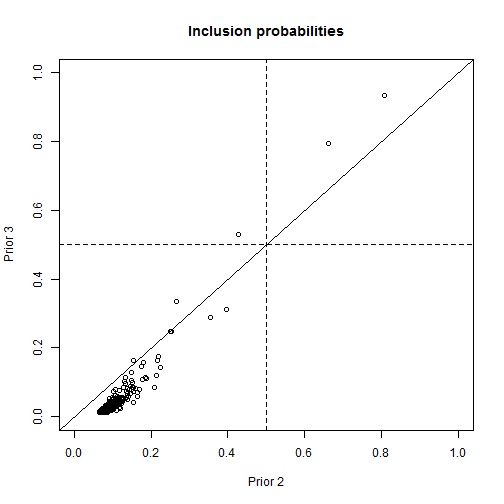quantile(p1[burn:iters],c(0.5,0.05,0.95))

## 50%  5% 95%
##  33  12 535

   quantile(p2[burn:iters],c(0.5,0.05,0.95))

## 50%  5% 95%
##  28  12 392

   quantile(p3[burn:iters],c(0.5,0.05,0.95))

## 50%  5% 95%
##  20  11  64


Summary:

Regardless of the prior, the same few covariates emerge as the most likely variables to be included in the model. However, there is some prior senstivity in terms of the number of variables included in the model and the subset of those with inclusion probability greater than 0.5.